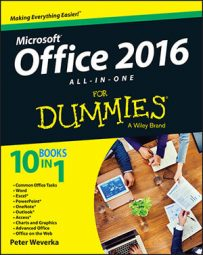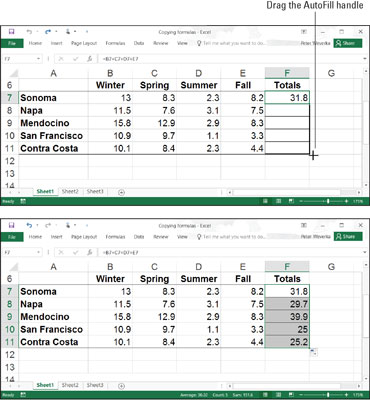##### Office 2016 All-in-One For DummiesOften in Excel 2016 worksheets, you use the same formula across a row or down a column, but different cell references are used. For example, in the worksheet shown here, column F totals the rainfall figures in rows 7 through 11. To enter formulas for totaling the rainfall figures in column F, you could laboriously enter formulas in cells F7, F8, F9, F10, and F11.

But a faster way is to enter the formula once in cell F7 and then copy the formula in F7 down the column to cells F8, F9, F10, and F11.Copying a formula.

When you copy a formula to a new cell, Excel adjusts the cell references in the formula so that the formula works in the cells to which it has been copied. Astounding! Opportunities to copy formulas abound on most worksheets. And copying formulas is the fastest and safest way to enter formulas in a worksheet.

Follow these steps to copy a formula:

1. Select the cell with the formula you want to copy down a column or across a row.

2. Drag the AutoFill handle across the cells to which you want to copy the formula.

This is the same AutoFill handle you drag to enter serial data. The AutoFill handle is the small green square in the lower-right corner of the cell. When you move the mouse pointer over it, it changes to a black cross.

3. Release the mouse button.

Click in the cells to which you copied the formula and glance at the Formula bar to make sure that the formula was copied correctly.

You can also copy formulas with the Copy and Paste commands. Just make sure that cell references refer correctly to the surrounding cells.